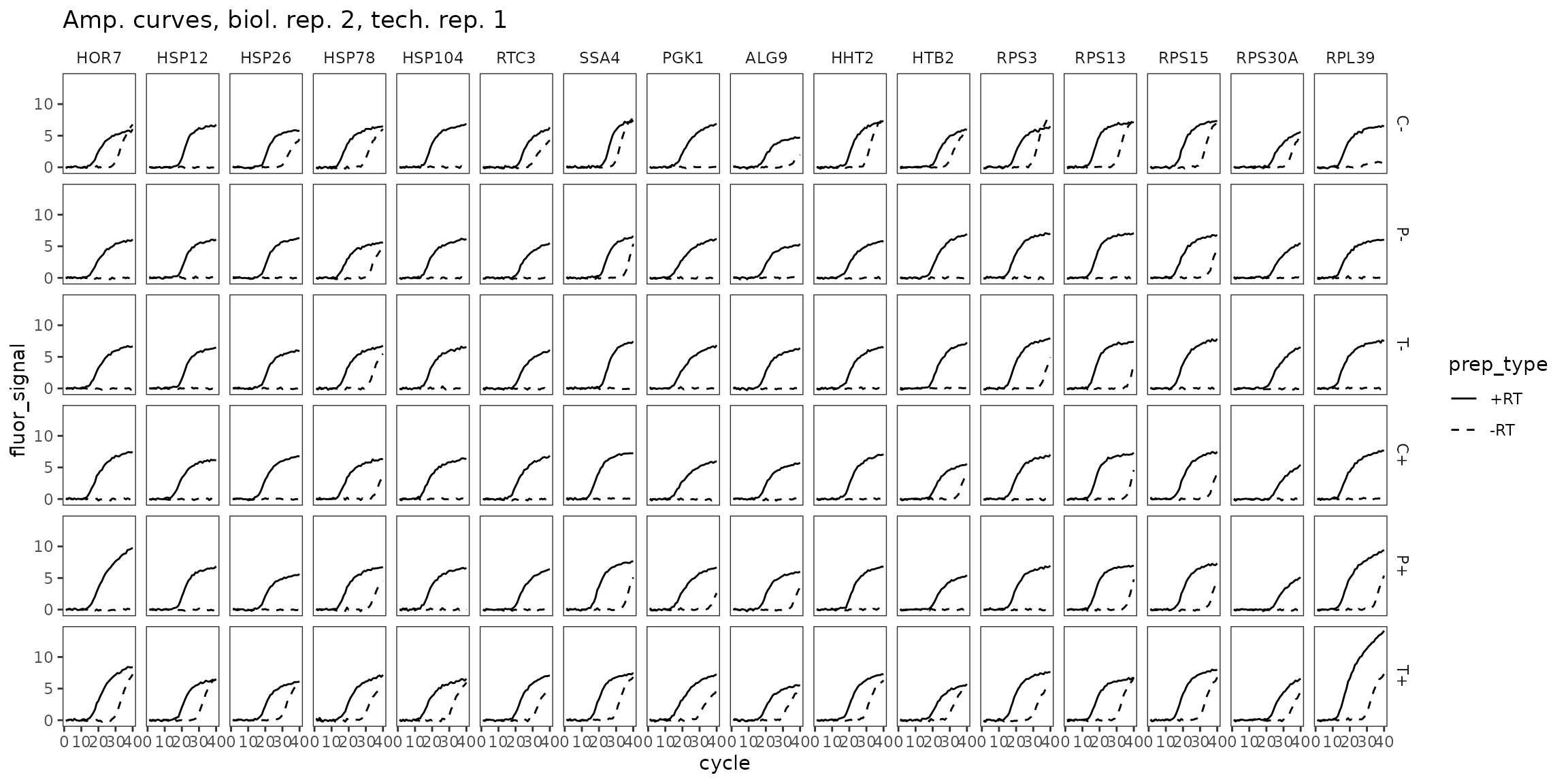## Summary: an example multifactorial qPCR experiment.

This vignette shows how to use tidyqpcr functions to normalize and plot data from multifactorial experiments: many primer sets, many conditions, two plates. This vignette is a more advanced example with complex data.

This is real RT-qPCR data by Edward Wallace in June 2018, testing the effect of heat shock and transcription-targeting drugs in Saccharomyces cerevisiae yeast.

### Pilot experiment

Do standard transcriptional inhibitors phenanthroline and thiolutin block the transcriptional heat shock response in yeast? This is a genuine question because some papers that argue that phenanthroline and thiolutin induce the transcriptional heat shock response.

Measure 16 primer sets: HOR7, HSP12, HSP26, HSP78, HSP104, RTC3, SSA4, PGK1, ALG9, HHT2, HTB2, RPS3, RPS13, RPS15, RPS30A, RPL39.

Test 6 conditions. That’s 3 transcriptional inhibitors (no drug control, 150ug/mL 1,10-phenanthroline, 3ug/mL thiolutin) in each of 2 conditions (- heat shock control, + heat shock 42C 10min), 2 biol reps each:

``````- *C-* Control -heat
- *P-* Phenanthroline -heat
- *T-* Thiolutin -heat
- *C+* Control +heat
- *P+* Phenanthroline +heat
- *T+* Thiolutin +heat``````

### Setup knitr options and load packages

``````# knitr options for report generation
knitr::opts_chunk\$set(
warning = FALSE, message = FALSE, echo = TRUE, cache = FALSE,
results = "show"
)

library(tidyr)
library(ggplot2)
library(dplyr)
library(tidyqpcr)

# set default theme for graphics
theme_set(theme_bw(base_size = 11) %+replace%
theme(
strip.background = element_blank()
))``````

### Label and plan plates

Reverse transcription by random primers mixed with oligo-dT.

``````# list target_ids of primer sets
target_id_levels <- c(
"HOR7", "HSP12", "HSP26", "HSP78",
"HSP104", "RTC3", "SSA4", "PGK1",
"ALG9", " HHT2", "HTB2", "RPS3",
"RPS13", "RPS15", "RPS30A", "RPL39"
)

rowkey <- tibble(
well_row = LETTERS[1:16],
target_id = factor(target_id_levels, levels = target_id_levels)
)

# Set up experimental samples
heat_levels <- c("-", "+")
heat_values <- factor(rep(heat_levels, each = 3), levels = heat_levels)
drug_levels <- c("C", "P", "T")
drug_values <- factor(rep(drug_levels, times = 2), levels = drug_levels)
condition_levels <- paste0(drug_levels, rep(heat_levels, each = 3))
condition_values <- factor(condition_levels, levels = condition_levels)

colkey <- create_colkey_6_in_24(
heat = heat_values,
drug = drug_values,
condition = condition_values
)

plateplan <-
label_plate_rowcol(
create_blank_plate(well_row = LETTERS[1:16], well_col = 1:24),
rowkey, colkey
)``````

Display the plate plan using display_plate_qpcr.

``````display_plate_qpcr(plateplan %>%
mutate(sample_id = condition))``````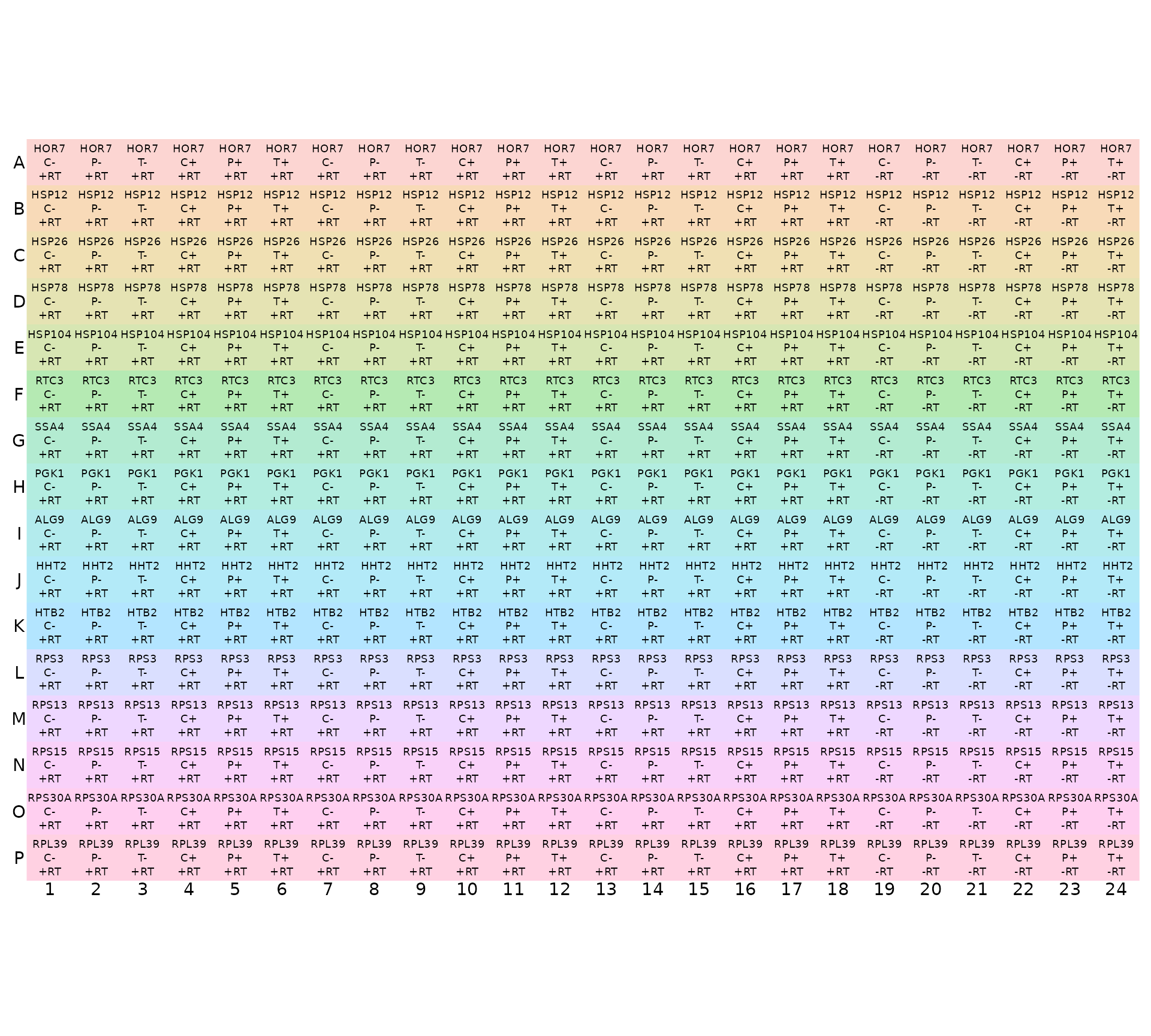Note that display_plate_qpcr requires a column called `sample_id`, which here we had to make from the `condition` variable using `mutate(sample_id=condition)`. The reason for doing this is that we have replicate samples of the same condition in different plates, and so we assign the unique sample name for each replicate after loading the plates together using `unite` in the next code chunk.

``````# read my plate data, one at a time, with biol_rep and plate number

# NOTE: system.file() accesses data from this R package
# To use your own data, remove the call to system.file(),
# or to another relevant read_ function

file_path_cq_plate1 <- system.file("extdata",
"Edward_qPCR_TxnInhibitors_HS_2018-06-15_plate1_Cq.txt.gz",
package = "tidyqpcr")

plate1 <- file_path_cq_plate1 %>%
left_join(plateplan, by = "well") %>%
mutate(biol_rep = "1", plate = "1")

file_path_cq_plate2 <- system.file("extdata",
"Edward_qPCR_TxnInhibitors_HS_2018-06-15_plate2_Cq.txt.gz",
package = "tidyqpcr")

plate2 <- file_path_cq_plate2 %>%
left_join(plateplan, by = "well") %>%
mutate(biol_rep = "2", plate = "2")

# combine data from both plates into a single data frame
plates <- bind_rows(plate1, plate2) %>%
unite(sample_id, condition, biol_rep, sep = "", remove = FALSE)

summary(plates)``````
``````##  include            color           well           sample_info
##  Mode:logical   Min.   :  255   Length:768         Length:768
##  TRUE:768       1st Qu.:  255   Class :character   Class :character
##                 Median :  255   Mode  :character   Mode  :character
##                 Mean   : 9738
##                 3rd Qu.:  255
##                 Max.   :65280
##
##        cq        concentration    standard  status           well_row
##  Min.   : 3.11   Min.   : NA   Min.   :0   Mode:logical   A      : 48
##  1st Qu.:15.92   1st Qu.: NA   1st Qu.:0   NA's:768       B      : 48
##  Median :18.12   Median : NA   Median :0                  C      : 48
##  Mean   :19.86   Mean   :NaN   Mean   :0                  D      : 48
##  3rd Qu.:21.19   3rd Qu.: NA   3rd Qu.:0                  E      : 48
##  Max.   :37.51   Max.   : NA   Max.   :0                  F      : 48
##  NA's   :112     NA's   :768                              (Other):480
##     well_col   prep_type tech_rep heat    drug     sample_id         condition
##  1      : 32   +RT:576   1:384    -:384   C:256   Length:768         C-:128
##  2      : 32   -RT:192   2:192    +:384   P:256   Class :character   P-:128
##  3      : 32             3:192            T:256   Mode  :character   T-:128
##  4      : 32                                                         C+:128
##  5      : 32                                                         P+:128
##  6      : 32                                                         T+:128
##  (Other):576
##    target_id     biol_rep            plate
##  HOR7   : 48   Length:768         Length:768
##  HSP12  : 48   Class :character   Class :character
##  HSP26  : 48   Mode  :character   Mode  :character
##  HSP78  : 48
##  HSP104 : 48
##  RTC3   : 48
##  (Other):480``````

## Plot unnormalized data

### -RT controls are low

``````ggplot(data = plates) +
geom_point(aes(x = target_id, y = cq, shape = condition, colour = condition),
position = position_jitter(width = 0.2, height = 0)
) +
labs(
y = "Cycle count to threshold",
title = "All reps, unnormalized"
) +
scale_shape_manual(values = c(15:18, 5:6)) +
facet_grid(biol_rep ~ prep_type) +
theme(
axis.text.x = element_text(angle = 90, vjust = 0.5),
panel.border = element_rect(
fill = NA, linetype = 1,
colour = "grey50", size = 0.5
)
)``````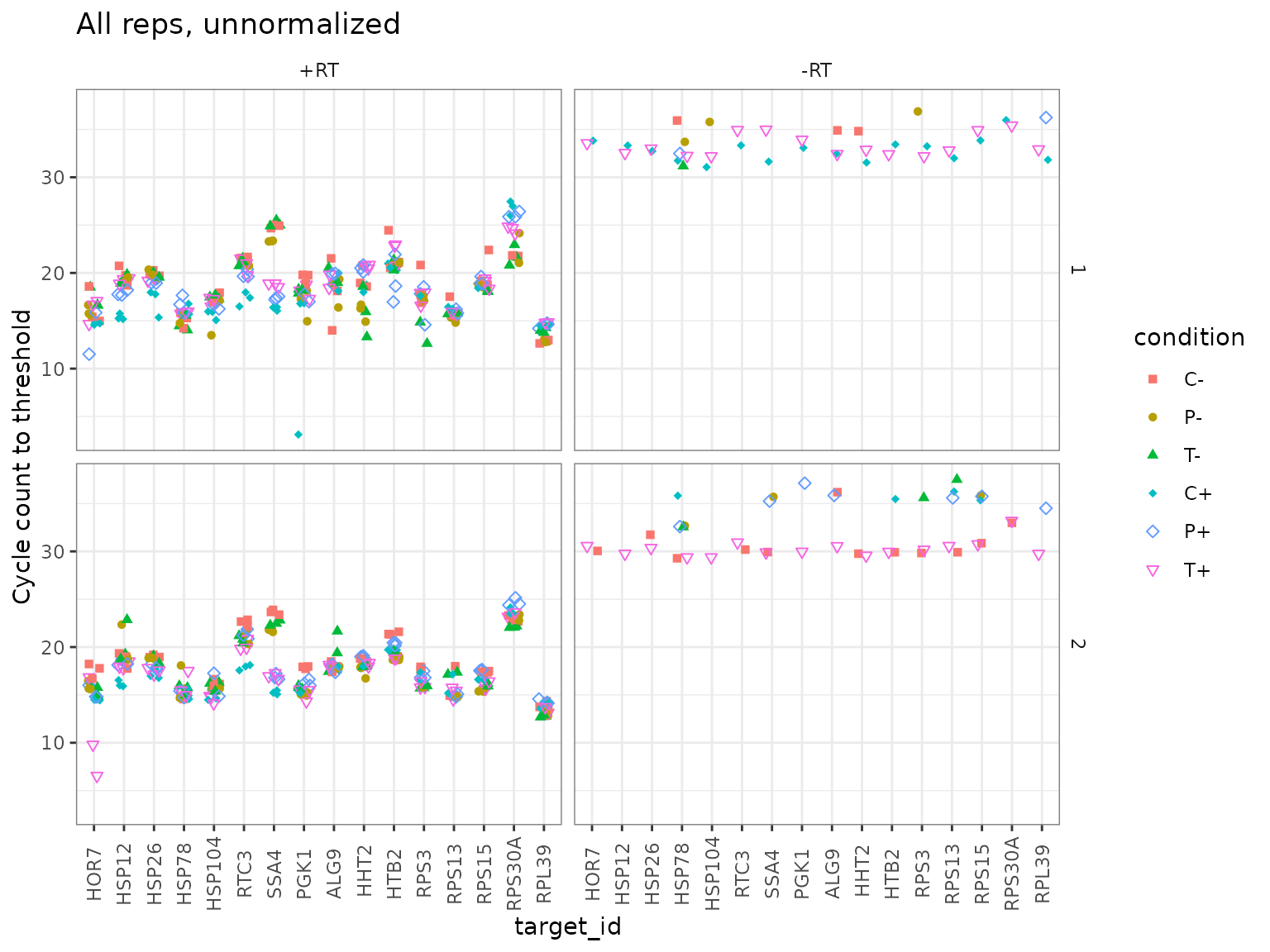### Normalize Cq to PGK1, within Sample only

``````platesnorm <- plates %>%
filter(prep_type == "+RT") %>%
calculate_deltacq_bysampleid(ref_target_ids = "PGK1")

platesmed <- platesnorm %>%
group_by(sample_id, condition, biol_rep, heat, drug, target_id) %>%
summarize(
delta_cq = median(delta_cq, na.rm = TRUE),
rel_abund = median(rel_abund, na.rm = TRUE)
)

filter(platesmed, target_id == "HSP26")``````
``````## # A tibble: 12 × 8
## # Groups:   sample_id, condition, biol_rep, heat, drug 
##    sample_id condition biol_rep heat  drug  target_id delta_cq rel_abund
##    <chr>     <fct>     <chr>    <fct> <fct> <fct>        <dbl>     <dbl>
##  1 C+1       C+        1        +     C     HSP26       0.98      0.507
##  2 C+2       C+        2        +     C     HSP26       1.58      0.334
##  3 C-1       C-        1        -     C     HSP26       0.0700    0.953
##  4 C-2       C-        2        -     C     HSP26       1.02      0.493
##  5 P+1       P+        1        +     P     HSP26       1.60      0.330
##  6 P+2       P+        2        +     P     HSP26       1.60      0.330
##  7 P-1       P-        1        -     P     HSP26       2.53      0.173
##  8 P-2       P-        2        -     P     HSP26       3.8       0.0718
##  9 T+1       T+        1        +     T     HSP26       1.19      0.441
## 10 T+2       T+        2        +     T     HSP26       2.06      0.240
## 11 T-1       T-        1        -     T     HSP26       1.44      0.369
## 12 T-2       T-        2        -     T     HSP26       3.02      0.123``````

### Plot normalized data, all reps

``````ggplot(data = platesnorm) +
geom_point(aes(x = target_id,
y = delta_cq,
shape = condition,
colour = condition),
position = position_jitter(width = 0.2, height = 0)
) +
labs(y = "Cq relative to PGK1") +
scale_shape_manual(values = c(15:18, 5:6)) +
facet_grid(biol_rep ~ .) +
theme(
axis.text.x = element_text(angle = 90, vjust = 0.5),
panel.border = element_rect(
fill = NA, linetype = 1,
colour = "grey50", size = 0.5
)
)``````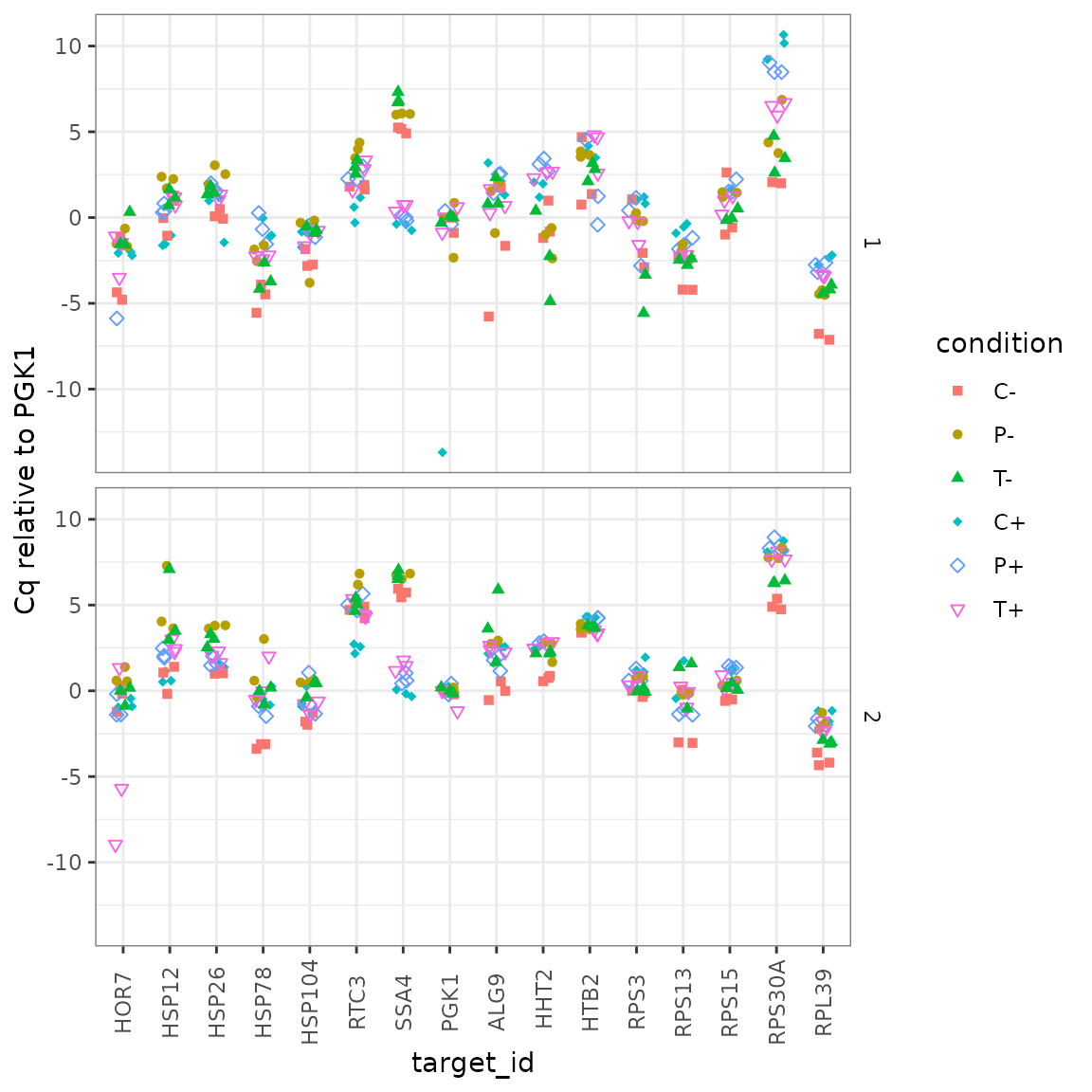### Plot normalized data, summarized vs target_id

#### All on same axes

This plot shows all the summarized data on the same axes, but it is hard to pick out the different conditions by eye.

``````ggplot(data = platesmed) +
geom_point(aes(x = target_id,
y = rel_abund,
shape = biol_rep,
colour = condition),
position = position_jitter(width = 0.2, height = 0)
) +
scale_shape_manual(values = c(15:18, 5:6)) +
scale_y_log10("mRNA relative detection",
labels = scales::label_number()) +
theme(axis.text.x = element_text(angle = 90, vjust = 0.5))``````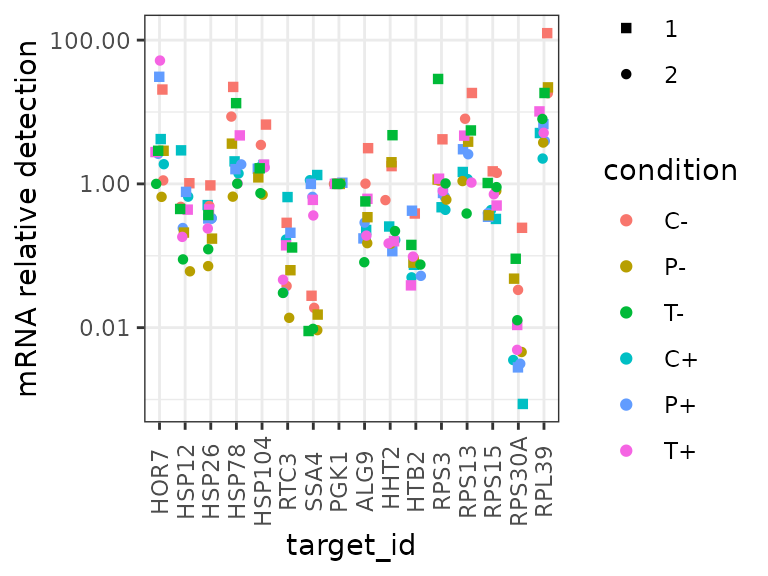#### Faceted by drug treatment

This plot shows all the summarized data “faceted” on different axes for different drug treatments. It highlights that, for example, SSA4 detection increases in response to heat in all drug treatments.

``````ggplot(data = platesmed) +
geom_point(aes(x = target_id, y = rel_abund, shape = biol_rep, colour = heat),
position = position_jitter(width = 0.2, height = 0)
) +
facet_wrap(~drug, ncol = 3) +
scale_colour_manual(values = c("-" = "grey50", "+" = "red")) +
scale_y_log10("mRNA relative detection",
labels = scales::label_number()) +
theme(axis.text.x = element_text(angle = 90, vjust = 0.5))``````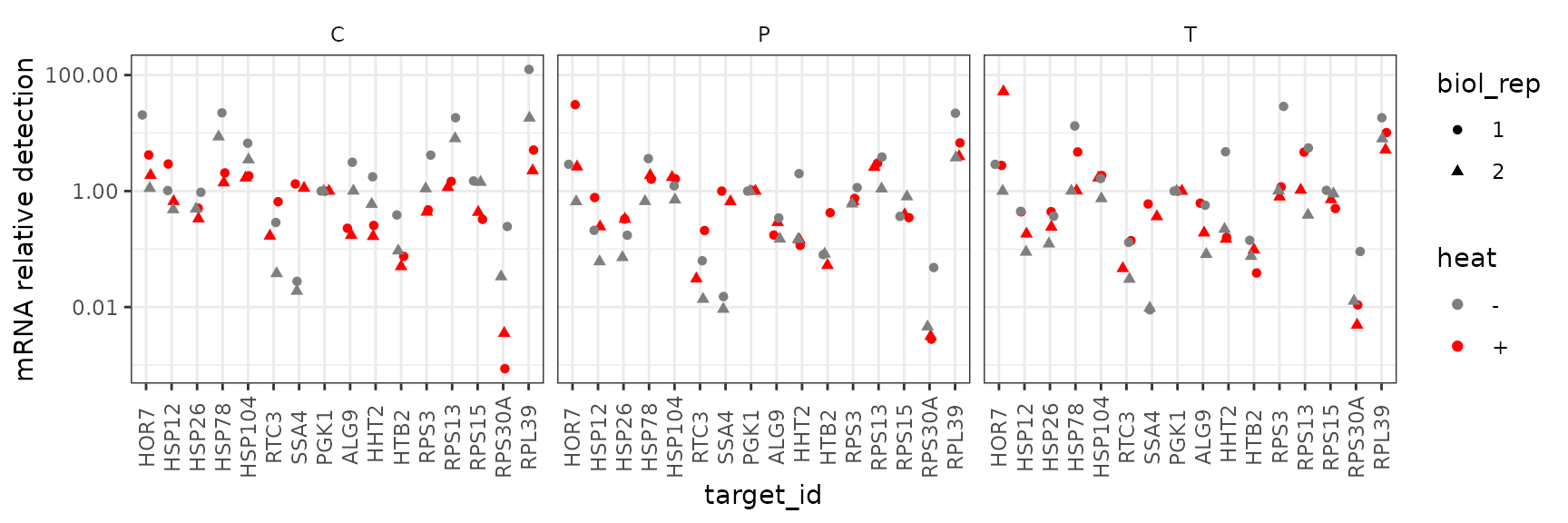#### Faceted by heat shock condition

By contrast, this plot shows all the summarized data “faceted” on different axes for different conditions. This shows that there is no clear response to the drug treatments in either condition.

``````ggplot(data = platesmed) +
geom_point(aes(x = target_id, y = rel_abund, shape = biol_rep, colour = drug),
position = position_jitter(width = 0.2, height = 0)
) +
facet_wrap(~heat, ncol = 3) +
scale_y_log10("mRNA relative detection",
labels = scales::label_number()) +
theme(axis.text.x = element_text(angle = 90, vjust = 0.5))``````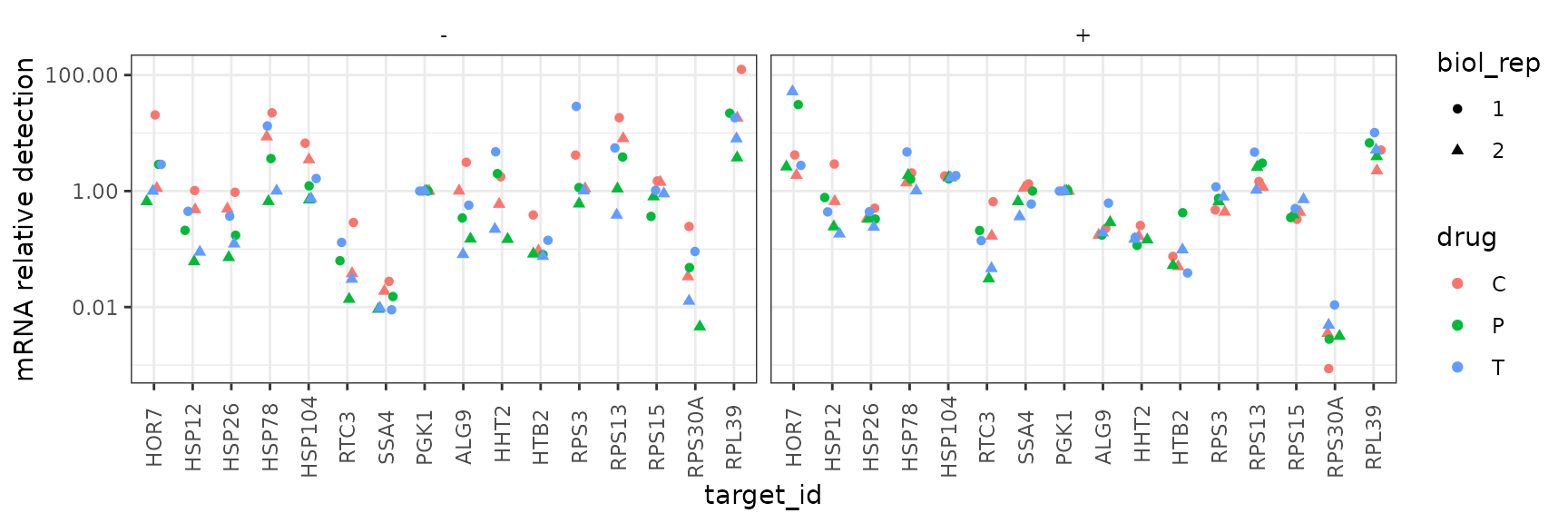## Melt and Amplification Curves

``````# NOTE: system.file() accesses data from this R package
# To use your own data, remove the call to system.file(),
# or to another relevant read_ function

file_path_raw_plate1 <- system.file("extdata/Edward_qPCR_TxnInhibitors_HS_2018-06-15_plate1.txt.gz",
package = "tidyqpcr")

plate1curve <- file_path_raw_plate1 %>%
debaseline() %>%
left_join(plateplan, by = "well") %>%
mutate(biol_rep = 1, plate = 1)

file_path_raw_plate2 <- system.file("extdata/Edward_qPCR_TxnInhibitors_HS_2018-06-15_plate2.txt.gz",
package = "tidyqpcr")

plate2curve <- file_path_raw_plate2 %>%
debaseline() %>%
left_join(plateplan, by = "well") %>%
mutate(biol_rep = 2, plate = 2)

platesamp <- bind_rows(plate1curve, plate2curve) %>%
filter(program_no == 2)

platesmelt <- bind_rows(plate1curve, plate2curve) %>%
filter(program_no == 3) %>%
calculate_drdt_plate() %>%
filter(temperature >= 61)``````

### Melt Curves, biol_rep 1

``````ggplot(
data = platesmelt %>%
filter(tech_rep == 1, biol_rep == 1),
aes(x = temperature, y = dRdT, linetype = prep_type)
) +
facet_grid(condition ~ target_id) +
geom_line() +
scale_linetype_manual(values = c("-RT" = "dashed", "+RT" = "solid")) +
scale_x_continuous(breaks = seq(60, 100, 10),
minor_breaks = seq(60, 100, 5)) +
labs(title = "Melt curves, biol. rep. 1, tech. rep. 1") +
theme(panel.grid = element_blank())``````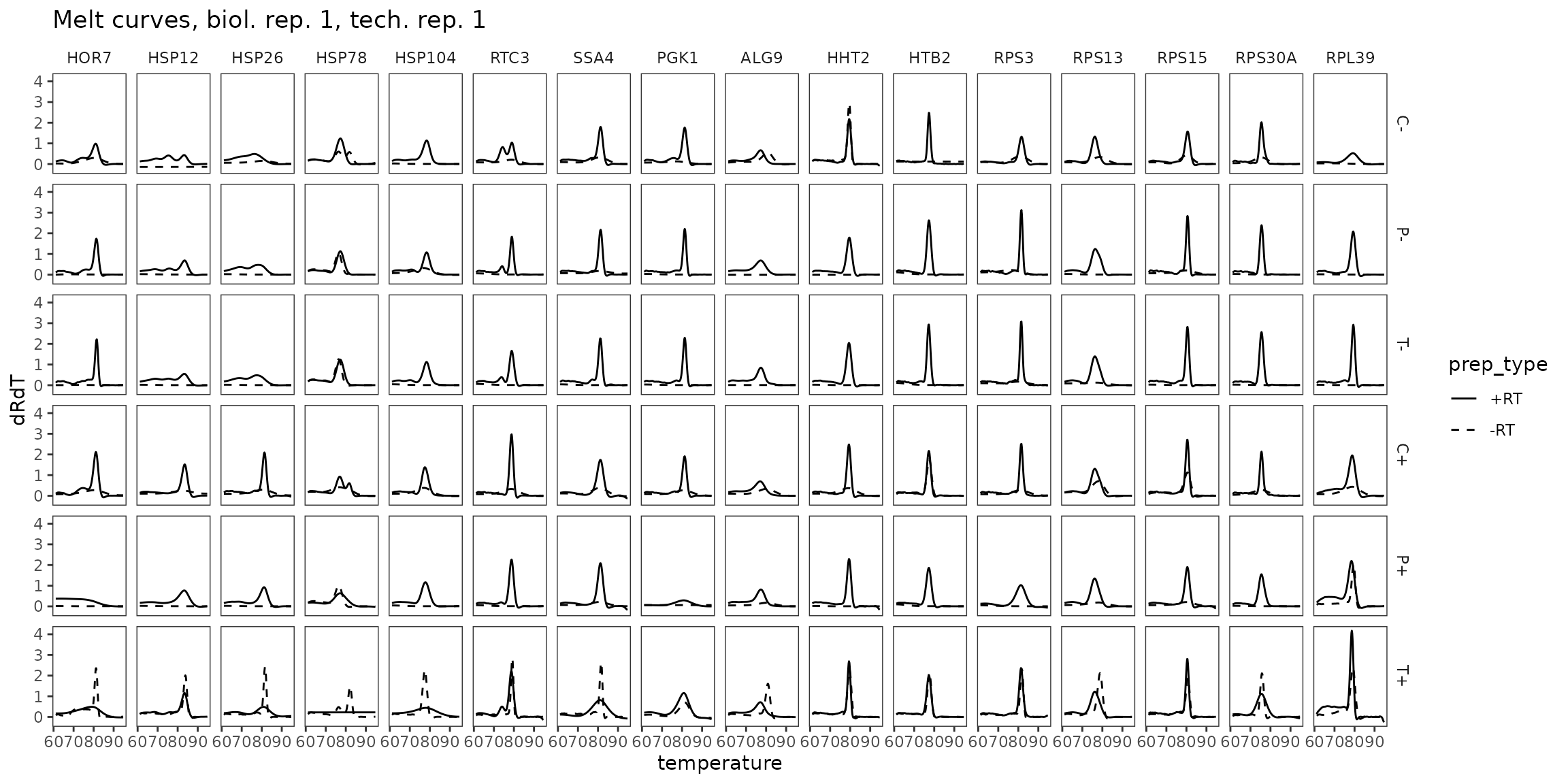### Melt Curves, biol_rep 2

``````ggplot(
data = platesmelt %>%
filter(tech_rep == 1, biol_rep == 2),
aes(x = temperature, y = dRdT, linetype = prep_type)
) +
facet_grid(condition ~ target_id) +
geom_line() +
scale_linetype_manual(values = c("-RT" = "dashed", "+RT" = "solid")) +
scale_x_continuous(breaks = seq(60, 100, 10),
minor_breaks = seq(60, 100, 5)) +
labs(title = "Melt curves, biol. rep. 2, tech. rep. 1") +
theme(panel.grid = element_blank())``````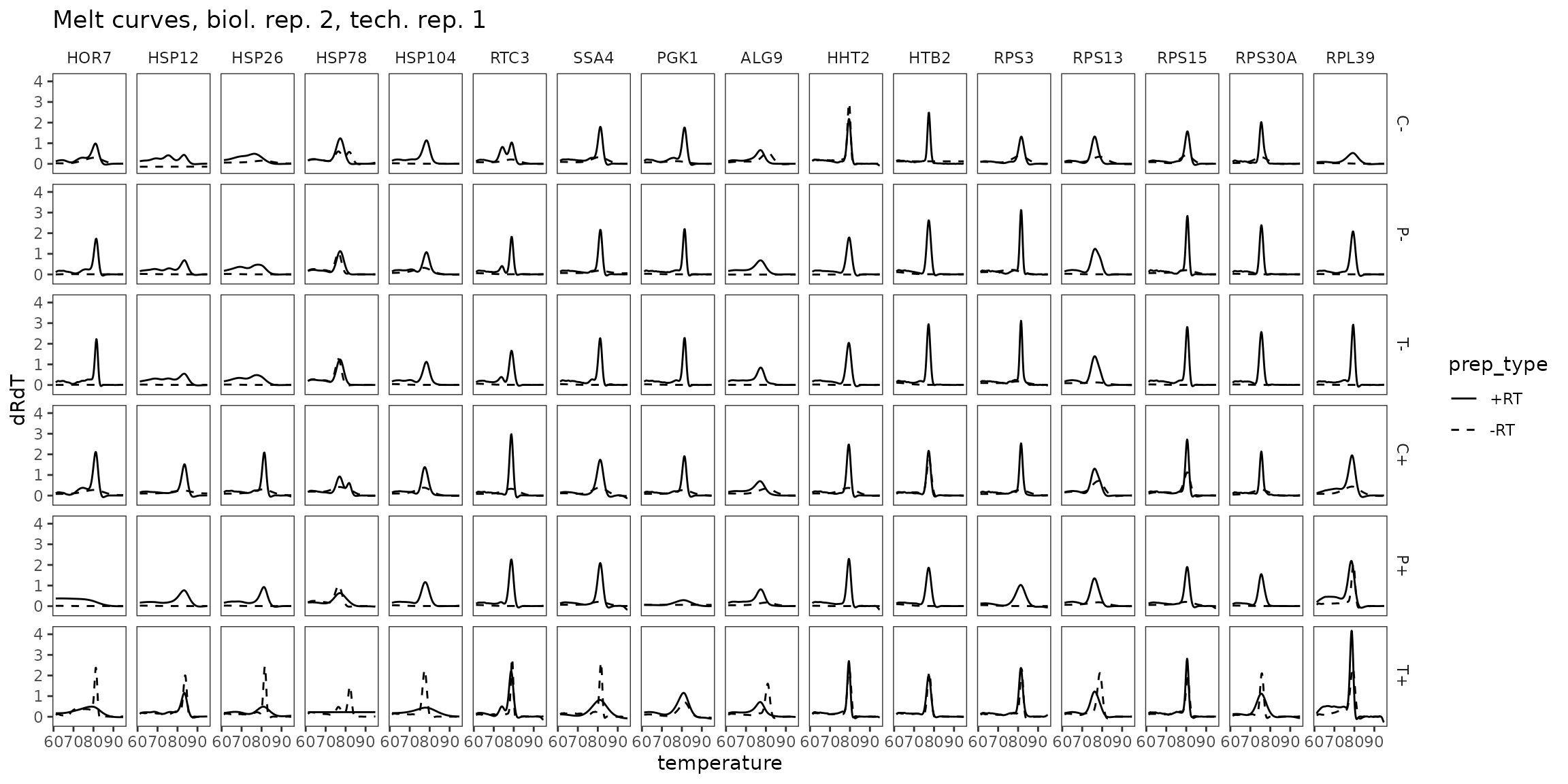### Amp Curves, biol_rep 1

``````ggplot(
data = platesamp %>%
filter(tech_rep == 1, biol_rep == 1),
aes(x = cycle, y = fluor_signal, linetype = prep_type)
) +
facet_grid(condition ~ target_id) +
geom_line() +
scale_linetype_manual(values = c("-RT" = "dashed", "+RT" = "solid")) +
expand_limits(y = 0) +
labs(title = "Amp. curves, biol. rep. 1, tech. rep. 1") +
theme(panel.grid = element_blank())``````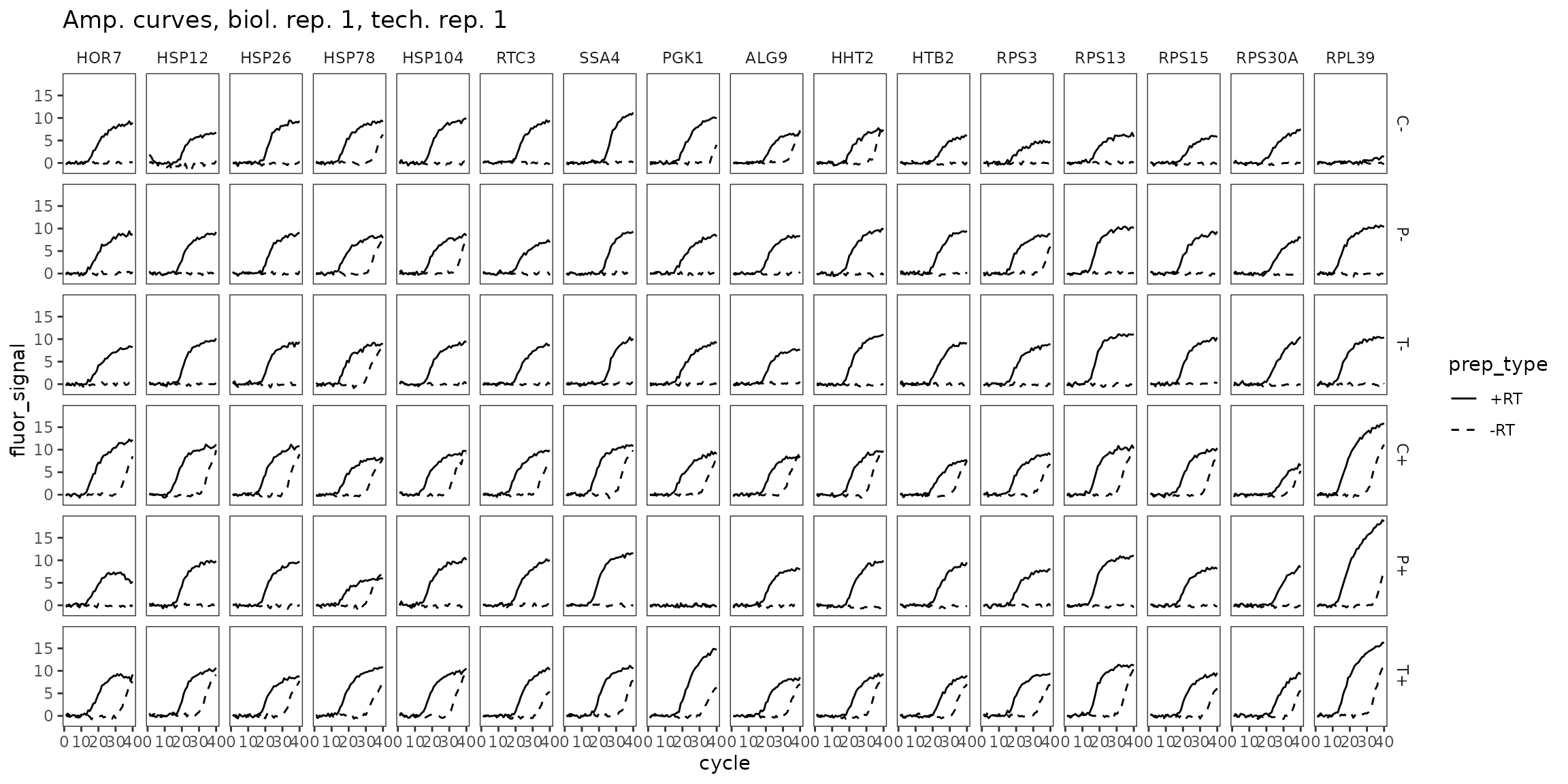``````ggplot(
data = platesamp %>%
filter(tech_rep == 1, biol_rep == 2),
aes(x = cycle, y = fluor_signal, linetype = prep_type)
) +
facet_grid(condition ~ target_id) +
geom_line() +
scale_linetype_manual(values = c("-RT" = "dashed", "+RT" = "solid")) +
expand_limits(y = 0) +
labs(title = "Amp. curves, biol. rep. 2, tech. rep. 1") +
theme(panel.grid = element_blank())``````